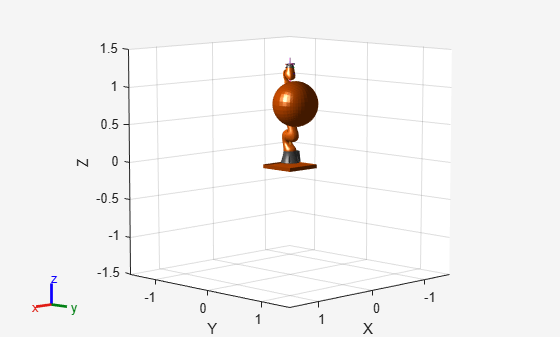Main Content

# plan

Plan path using RRT for manipulators

## Syntax

``path = plan(rrt,startConfig,goalConfig)``
``path = plan(rrt,startConfig,goalRegion)``
``[path,solnInfo] = plan(___)``

## Description

example

````path = plan(rrt,startConfig,goalConfig)` plans a path between the specified start and goal configurations using the manipulator rapidly exploring random trees (RRT) planner `rrt`.```

example

````path = plan(rrt,startConfig,goalRegion)` plans a path between the specified start and a goal region as a `workspaceGoalRegion` object```
````[path,solnInfo] = plan(___)` also returns solution info about the results from the RRT planner using the previous input arguments.```

## Examples

collapse all

Use the `manipulatorRRT` object to plan a path for a rigid body tree robot model in an environment with obstacles. Visualize the planned path with interpolated states.

Load a robot model into the workspace. Use the KUKA LBR iiwa© manipulator arm.

`robot = loadrobot("kukaIiwa14","DataFormat","row");`

Generate the environment for the robot. Create collision objects and specify their poses relative to the robot base. Visualize the environment.

```env = {collisionBox(0.5, 0.5, 0.05) collisionSphere(0.3)}; env{1}.Pose(3, end) = -0.05; env{2}.Pose(1:3, end) = [0.1 0.2 0.8]; show(robot); hold on show(env{1}) show(env{2})```Create the RRT planner for the robot model.

`rrt = manipulatorRRT(robot,env);`

Specify a start and a goal configuration.

```startConfig = [0.08 -0.65 0.05 0.02 0.04 0.49 0.04]; goalConfig = [2.97 -1.05 0.05 0.02 0.04 0.49 0.04];```

Plan the path. Due to the randomness of the RRT algorithm, set the `rng` seed for repeatability.

```rng(0) path = plan(rrt,startConfig,goalConfig);```

Visualize the path. To add more intermediate states, interpolate the path. By default, the `interpolate` object function uses the value of `ValidationDistance` property to determine the number of intermediate states. The `for` loop shows every 20th element of the interpolated path.

```interpPath = interpolate(rrt,path); clf for i = 1:20:size(interpPath,1) show(robot,interpPath(i,:)); hold on end show(env{1}) show(env{2}) hold off```Specify a goal region in your workspace and plan a path within those bounds. The `workspaceGoalRegion` object defines the bounds on the XYZ-position and ZYX Euler orientation of the robot end effector. The `manipulatorRRT` object plans a path based on that goal region and samples random poses within the bounds.

Load an existing robot model as a `rigidBodyTree` object.

```robot = loadrobot("kinovaGen3", "DataFormat", "row"); ax = show(robot);```Create Path Planner

Create a rapidly-exploring random tree (RRT) path planner for the robot. This example uses an empty environment, but this workflow also works well with cluttered environments. You can add collision objects to the environment like the `collisionBox` or `collisionMesh` object.

`planner = manipulatorRRT(robot,{});`

Define Goal Region

Create a workspace goal region using the end-effector body name of the robot.

Define the goal region parameters for your workspace. The goal region includes a reference pose, XYZ-position bounds, and orientation limits on the ZYX Euler angles. This example specifies bounds on the XY-plane in meters and allows rotation about the Z-axis in radians.

```goalRegion = workspaceGoalRegion(robot.BodyNames{end}); goalRegion.ReferencePose = trvec2tform([0.5 0.5 0.2]); goalRegion.Bounds(1, :) = [-0.2 0.2]; % X Bounds goalRegion.Bounds(2, :) = [-0.2 0.2]; % Y Bounds goalRegion.Bounds(4, :) = [-pi/2 pi/2]; % Rotation about the Z-axis```

You can also apply a fixed offset to all poses sampled within the region. This offset can account for grasping tools or variations in dimensions within your workspace. For this example, apply a fixed transformation that places the end effector 5 cm above the workspace.

```goalRegion.EndEffectorOffsetPose = trvec2tform([0 0 0.05]); hold on show(goalRegion);```Plan Path To Goal Region

Plan a path to the goal region from the robot's home configuration. Due to the randomness in the RRT algorithm, this example sets the `rng` seed to ensure repeatable results.

```rng(0) path = plan(planner,homeConfiguration(robot),goalRegion);```

Show the robot executing the path. To visualize a more realistic path, interpolate points between path configurations.

```interpConfigurations = interpolate(planner,path,5); for i = 1 : size(interpConfigurations) show(robot,interpConfigurations(i,:),"PreservePlot",false); set(ax,'ZLim',[-0.05 0.75],'YLim',[-0.05 1],'XLim',[-0.05 1],... 'CameraViewAngle',5) drawnow end hold off```Adjust End-effector Pose

Notice that the robot arm approaches the workspace from the bottom. To flip the orientation of the final position, add a `pi` rotation to the Y-axis for the reference pose.

```goalRegion.EndEffectorOffsetPose = ... goalRegion.EndEffectorOffsetPose*eul2tform([0 pi 0],"ZYX");```

Replan the path and visualize the robot motion again. The robot now approaches from the top.

```hold on show(goalRegion); path = plan(planner,homeConfiguration(robot),goalRegion); interpConfigurations = interpolate(planner,path,5); for i = 1 : size(interpConfigurations) show(robot, interpConfigurations(i, :),"PreservePlot",false); set(ax,'ZLim',[-0.05 0.75],'YLim',[-0.05 1],'XLim',[-0.05 1]) drawnow; end hold off```## Input Arguments

collapse all

Manipulator RRT planner, specified as a `manipulatorRRT` object. This planner is for a specific rigid body tree robot model stored as a `rigidBodyTree` object.

Initial robot configuration, specified as an n-element vector of joint positions for the `rigidBodyTree` object stored in the RRT planner `rrt`. n is the number of nonfixed joints in the robot model.

Data Types: `double`

Desired robot configuration, specified as an n-element vector of joint positions for the `rigidBodyTree` object stored in the RRT planner `rrt`. n is the number of nonfixed joints in the robot model.

Data Types: `double`

Workspace goal region, specified as a `workspaceGoalRegion` object.

The `workspaceGoalRegion` object defines the bounds on the end-effector pose and the `sample` object function returns random poses to add to the RRT tree. Set the WorkspaceGoalRegionBias property to change the probability of sampling a state within the goal region.

## Output Arguments

collapse all

Planned path in joint space, returned as an r-by-n matrix of joint configurations, where r is the number of configurations in the path, and n is the number of nonfixed joints in the `rigidBodyTree` robot model.

Data Types: `double`

Solution information from planner, returned as a structure with these fields:

• `IsPathFound` — A logical indicating if a path was found

• `ExitFlag` — An integer indicating why the planner terminated:

• `1` — Goal configuration reached

• `2` — Maximum number of iterations reached

Data Types: `struct`

## Extended Capabilities

### C/C++ Code GenerationGenerate C and C++ code using MATLAB® Coder™.

Introduced in R2020b